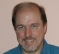## What is the significance of whitening "signal + noise" at the receiver side in a wireless communication systemStarted by 6 years ago7 replieslatest reply 6 years ago2316 views

Hi,

I came across this process when working on a wireless communication project where the whitening process is applied before we do signal detection, but I found little clue why we need to do this. Most of the text book/online material explains "how" to do so but gave little insight on "why". Can someone explain what is the significance of doing so before signal detection and what is the consequence if we skip this stage?

Thanks,

william

[ - ]From what I know, the received signal in wireless system is y=hx + n. where n is complex gaussian white noise. After applying equalization, y* = y/h = x + n/h. The noise n/h is now no longer white but 'coloured' as the Power spectrum is not flat now. The ML decoding cannot be applied now since noise is not white. In order to compensate for this, we apply whitening on the noise so that ML decoding can be applied.

[ - ]Many detection and estimation formulations assume that the added noise is white (see "minimum variance" and "maximum likelihood" estimation).  When the noise is not white, we generally try to flatten its spectrum as much as we can, pushing its correlation closer to white. See https://en.wikipedia.org/wiki/Generalized_least_sq...

[ - ]but when we do whitening on "signal + noise", wouldn't we skew the signal to be detected as well? I am looking for what the side effect this could cause... (The whitening matrix is calculated from the time duration when we have "reference signal + noise" and it is then applied to the later time duration when we have " data signal+noise" before signal detection.)

[ - ]Yes, only the noise should be whitened.  In audio, we typically use segments of silence to form a noise-floor estimate.  Otherwise, you can try to preserve the spectrum where there is signal (i.e., don't whiten there).  In sinusoidal modeling, we sometimes extrapolate the noise across the gaps created by "removing" sinusoidal peaks to get a noise-floor estimate ("sines plus noise synthesis").

[ - ]